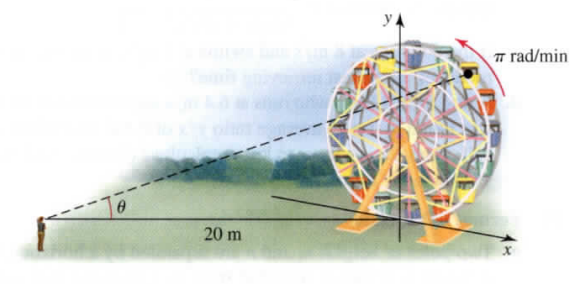×
Get Full Access to Calculus: Early Transcendentals - 1 Edition - Chapter 4.4 - Problem 43e
Get Full Access to Calculus: Early Transcendentals - 1 Edition - Chapter 4.4 - Problem 43e

×

# Solved: Watching a Ferris wheel An observer stands 20 mISBN: 9780321570567 2

## Solution for problem 43E Chapter 4.4

Calculus: Early Transcendentals | 1st Edition

• Textbook Solutions
• 2901 Step-by-step solutions solved by professors and subject experts
• Get 24/7 help from StudySoup virtual teaching assistantsCalculus: Early Transcendentals | 1st Edition

4 5 1 336 Reviews
28
1
Problem 43E

An observer stands 20 m from the bottom of a Ferris wheel on a line that is perpendicular to the face of the wheel, with her eyes at the level of the bottom of the wheel. The wheel revolves at a rate of $$\pi$$ rad/min and the observer's line of sight with a specific seat on the Ferris wheel makes an angle $$\theta$$ with the horizontal (see figure). At what time during a full revolution is $$\theta$$ changing most rapidly?Step-by-Step Solution:

Solution 43E Step 1: Consider that an observer stands 20 m from the bottom of the Ferris wheel on a line that is perpendicular to the face of the wheel. Consider the line with observer sight and a seat makes angle and the line length is L m. Now, consider the diameter of the wheel is r m. Also, consider that the radius of the wheel is r m. Now, the revolving rate is So, the height of the seat from the ground is: Here t is the time in minute. Draw the wheel accordingly

Step 2 of 4

Step 3 of 4

##### ISBN: 9780321570567

The full step-by-step solution to problem: 43E from chapter: 4.4 was answered by , our top Calculus solution expert on 03/03/17, 03:45PM. This textbook survival guide was created for the textbook: Calculus: Early Transcendentals, edition: 1. This full solution covers the following key subjects: wheel, ferris, observer, line, most. This expansive textbook survival guide covers 112 chapters, and 7700 solutions. Calculus: Early Transcendentals was written by and is associated to the ISBN: 9780321570567. The answer to “?An observer stands 20 m from the bottom of a Ferris wheel on a line that is perpendicular to the face of the wheel, with her eyes at the level of the bottom of the wheel. The wheel revolves at a rate of $$\pi$$ rad/min and the observer's line of sight with a specific seat on the Ferris wheel makes an angle $$\theta$$ with the horizontal (see figure). At what time during a full revolution is $$\theta$$ changing most rapidly?” is broken down into a number of easy to follow steps, and 80 words. Since the solution to 43E from 4.4 chapter was answered, more than 549 students have viewed the full step-by-step answer.

## Discover and learn what students are asking

Statistics: Informed Decisions Using Data : Introduction to the Practice of Statistics
?____________ are the characteristics of the individuals of the population being studied

Statistics: Informed Decisions Using Data : Discrete Probability Distributions
?State the condition required to use the Empirical Rule to check for unusual observations in a binomial experiment.

Statistics: Informed Decisions Using Data : Tests for Independence and the Homogeneity of Proportions
?Political Affiliation In the Sullivan Statistics Survey, respondents were asked to disclose their political affiliation (Democrat, Independent, Republ

Statistics: Informed Decisions Using Data : Comparing Three or More Means (One-Way Analysis of Variance)
?Reaction Time In an online psychology experiment sponsored by the University of Mississippi, researchers asked study participants to respond to variou

Unlock Textbook Solution

Enter your email below to unlock your verified solution to:

Solved: Watching a Ferris wheel An observer stands 20 m